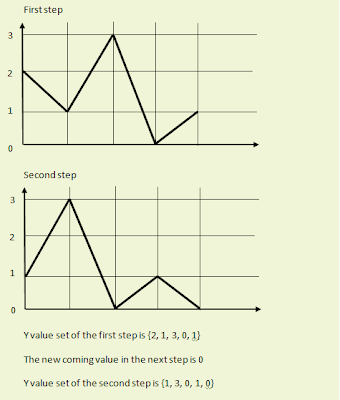## Monday, November 09, 2009

### Code Plugin for Blogger - How to Install Syntaxhighlighter

I found a nice blog post explaining how to install Syntaxhighlighter code plugin to blogger. It is very simple and works great.

1. If your browser is Firefox, you should add the styles manually
* copy the stylesheet at the location http://syntaxhighlighter.googlecode.com/svn/trunk/Styles/SyntaxHighlighter.css
* Go to Layout --> Edit HTML
* Paste the stylesheet before ]]> tag
* Save the template

2. Other relevant scripts (Other than Cpp) are located here

## Wednesday, November 04, 2009

### How to Plot Moving Graphs Using Flot Library

Plotting a moving graph is nothing other than plotting an instance of a varying data set at each refresh. However, to get the moving effect you need to change the data set as stated below

1. Discard the leftmost Y value of the previous step
2. Shift the remaining Y values to the left
3. Add the new coming value as the rightmost Y valueFlot is an opensource Javascript plotting library for jQuery. Now let's see how to do this using Flot.

1.jquery.js
2.jquery.flot.js
3.excanvas.js (This is needed if your browser is IE)

Now let's create the html page

```<html>
<!--Include the css file and flot libs-->

<script language="javascript" type="text/javascript" src="js/jquery.js"></script>
<script language="javascript" type="text/javascript" src="js/jquery.flot.js"></script>
<script language="javascript" type="text/javascript" src="js/excanvas.js"></script>
....
....
.... ```

Here is the CSS file used

```body {
font-family: sans-serif;
font-size: 16px;
margin: 50px;
max-width: 800px;
background-color:#000000;
}

div.graph {
width:500px;
height:300px;
}
```

Let's include javascript functions inside HEAD

```....
....
<script id="source" language="javascript" type="text/javascript">
var running = false;
var array;
var xscale = 100;

//this function does the plotting
function draw() {
//shift the values to left
for (var i = 0; i < this.xscale - 1; i++) {
this.array[i] = [i,this.array[i + 1]];  // (x,y)
}

//add the new coming value to the last postion
this.array[this.xscale - 1] = [this.xscale - 1,Math.random()];

\$.plot(\$("#graphdiv"), [
{
label: "Y vs X",
data: this.array,
lines: { show: true, fill: true, fillColor: "rgba(249, 28, 61,0.3)",lineWidth: 3.5 },
color:"rgba(249, 28, 61,1)"
}
],
{
xaxis: {
ticks: generateTicks(),
min: 0

},
yaxis: {
ticks: [0 , 0.2, 0.4, 0.6, 0.8, 1.0, 1.2],
min: 0
},

//placing a grid
grid: {
show: true,
color: '#474747',
tickColor: '#474747',
borderWidth: 1,
autoHighlight: true,
}

});
}

//This creates the data array with 0.0 inital Y values at the initialization time
function initialize() {
this.array = new Array();
for (var i = 0; i < xscale; i++) {
this.array[i] = [i, 0.0];
}
}

//This is used to generate ticks for X axis (value 0 will be on the right)
function generateTicks() {
var tickArray = [];
var startTick = 20;
var i = startTick - 1;
var weight = this.xscale / 20;
do {
var t = (startTick - i) * weight - 1;
var v = i * weight;
if (v == 0) {
v = "0";
}
tickArray.push([t, v]);
i--;
} while (i > -1);
return tickArray;
}

//This function is called once per every 1000ms
function refreshStat() {
if (!running) {
running = true;
draw();
running = false;
}
}

initialize();
refreshStat();
setInterval("refreshStat()", 1000);
});

</script>
```

Now here goes the BODY of the html

```<body>
<table cellspacing="50">
<tr>
<td>
<div id="graphdiv" class="graph"></div>
</td>
</tr>
</table>
</body>
</html>
```

Then you'll get a moving graph. I've posted some still instances of the graph below

You can customize the graphs as your wish. Flot API will help you on that. This is pretty simple and straight. Just give a try.